# RD Sharma Solutions Chapter 14 Coordinate Geometry Exercise 14.5 Class 10 Maths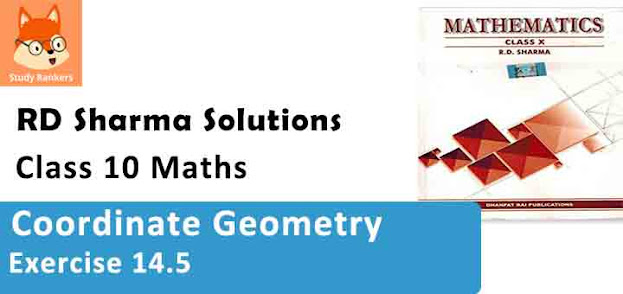Chapter Name RD Sharma Chapter 14 Coordinate Geometry Book Name RD Sharma Mathematics for Class 10 Other Exercises Exercise 14.1Exercise 14.2Exercise 14.3Exercise 14.4Exercise 14.5VSAQs and MCQs Related Study NCERT Solutions for Class 10 Maths

### Exercise 14.5 Solutions

1. Find the area of a triangle whose vertices are :

(i) (6, 3), (-3, 5) and (4, -2)
(ii) (at12, 2at1), (at22, 2at2) and (at32, 2at3)
(iii) (a, c + a), (a, c) and (-a, c – a)

Solution

(i) Co-ordinates of ∆ABC are A (6, 3), B (-3, 5) and C (4, -2)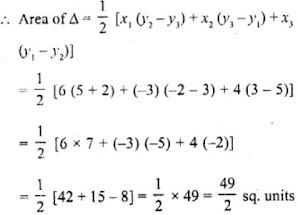(ii) Coordinates of ABC are A(at12, 2at1), B(at22, 2at2) and C(at32, 2at3)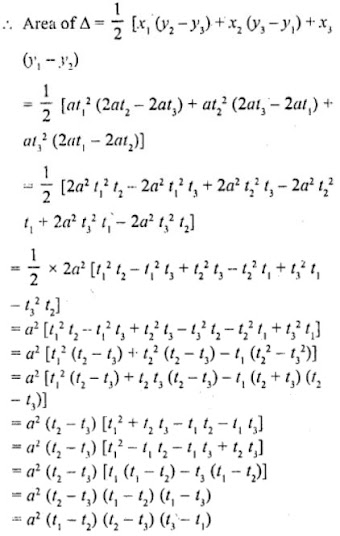(iii) Coordinates of △ABC are (a, c+a), (a, c) and (-a, c-a)
∴ Area of △ABC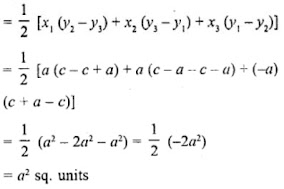2. Find the area of the quadrilaterals, the coordinates of whose vertices are
(i) (-3, 2), (5, 4), (7, -6) and (-5, -4)
(ii) (1, 2), (6, 2), (5, 3) and (3, 4)
(iii) (-4, -2), (-3, -5), (3, -2), (2, 3)

Solution

(i) Let vertices of quadrilateral ABCD be A (-3, 2), B (5, 4), C (7, -6) and D (-5, -4)
Join AC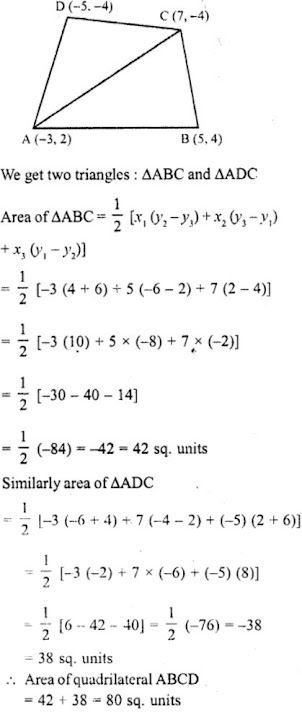(ii) Let the vertices of quadrilateral ABCD be A(1, 2), B(6, 2), C(5, 3) and D(3, 4).
Join AC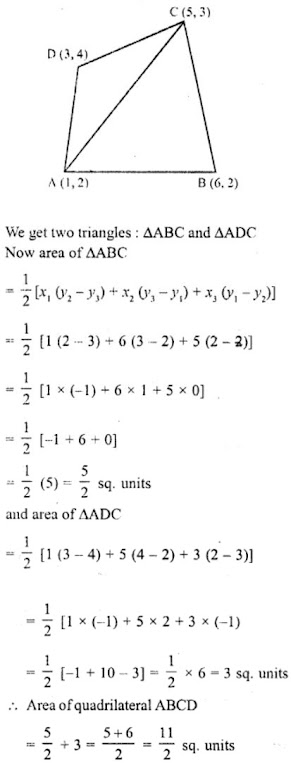(iii) Area of the quadrilateral ABCD whose coordinates are A(-4, -2), B(-3, -5), C(3, -2) and D(2, 3).
Join the diagonal AC which divides the quadrilateral ABCD into the triangle ABC and ACD.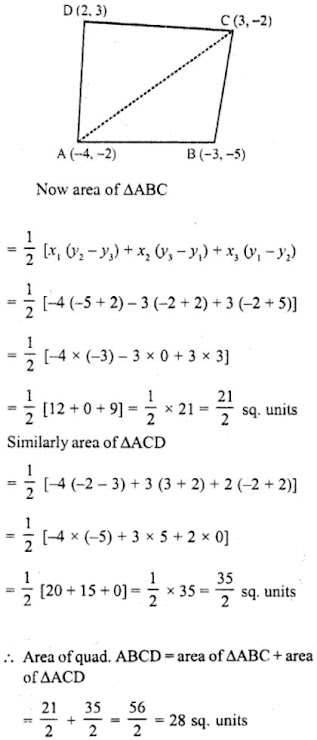3. The four vertices of a quadrilaterals are (1, 2), (-5, 6), (7, -4) and (k, -2) taken in order. If the area of the quadrilateral is zero, find the value of k ?

Solution

Let the vertices of quadrilateral ABCD be A (1, 2), B (-5, 6), C (7, -4) and D (k, -2).
Join AC.
We get two triangles ABC and ADC.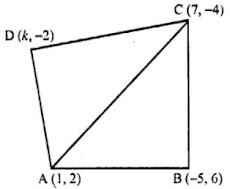Now, area of Δ∆ABC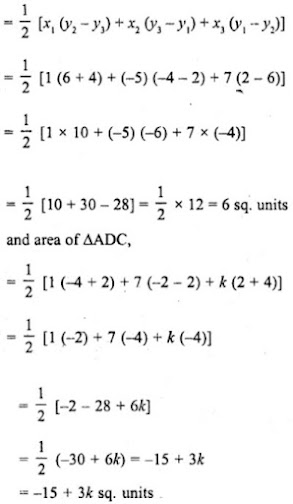Area of quadrilateral ABCD = (6 - 15 + 3k)
= (-9 + 3k) sq. units
But area of quadrilateral ABCD = 0 (given)
-9 + 3k = 0
⇒ 3k = 9
⇒ k = 9/3
⇒ k = 3

4. The vertices of ∆ABC are (-2, 1), (5, 4) and (2, -3) respectively. Find the area of the triangle and the length of the altitude through A.

Solution

Vertices of ∆ABC are A (-2, 1), B (5, 4) and C (2, -3) and AD ⊥ BC, let AD = h
Now area of ∆ABC,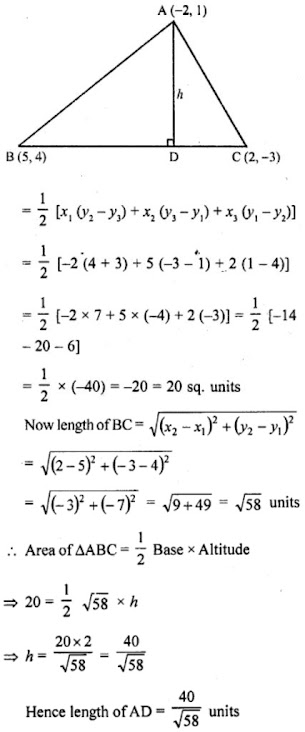5. Show that the following sets of points are collinear
(a) (2, 5), (4, 6) and (8, 8)
(b) (1, -1), (2, 1) and (4, 5)

Solution

We know that points are collinear if the area of the triangle formed by them is zero
(a) Vertices of ∆ABC are A(2, 5), B(4, 6) and C(8, 8)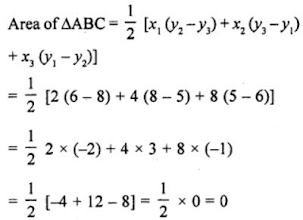∵ Area of ∆ABC = 0
∴ Points A, B and C are collinear.

(b) Vertices of ∆ABC are A(1, -1), B(2, 1) and C(4, 5)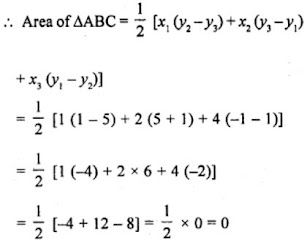∵ Area of ∆ABC = 0
∴ Points A, B and C are collinear.

6. Find the area of a quadrilateral ABCD, the coordinates of whose varieties are A (-3, 2), B (5, 4), C (7, -6) and D (-5, -4).

Solution

= area of ∆ABC + area of ∆ACD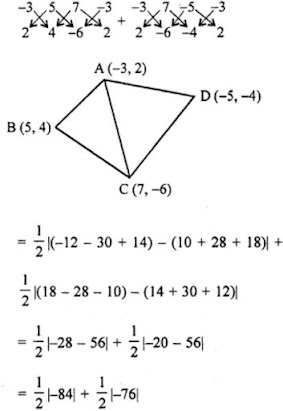= 42 + 38 = 80 sq. units
∴ Area of quadrilateral = 80 sq. units

7. In ∆ABC, the coordinates of vertex A are (0, -1) and D (1, 0) and E (0, 1) respectively the mid-points of the sides AB and AC. If F is the mid-point of side C, find the area of ∆DEF.

Solution

Let B (p, q), C (r, s) and F (x, y)
Mid-point of AB = Coordinates of D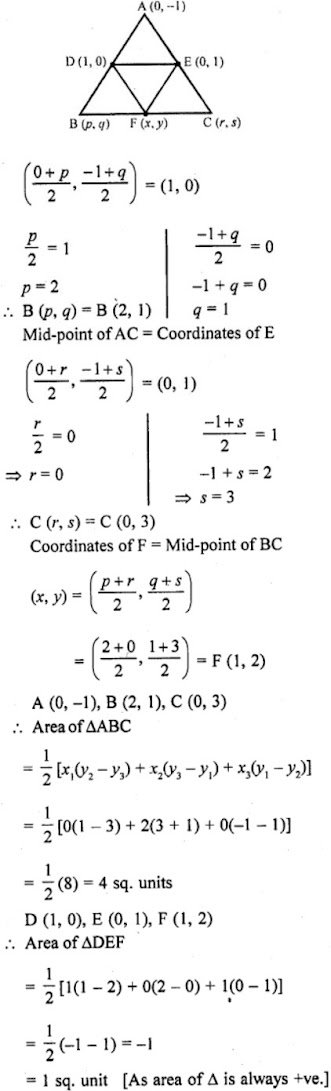8. Find the area of the triangle PQR with Q (3, 2) and the mid-points of the sides through Q being (2, -1) and (1, 2).

Solution

In ∆PQR, L and N are mid points of QR and QP respectively coordinates of Q are (3, 2) of L are (2, -1) and of N are (1, 2).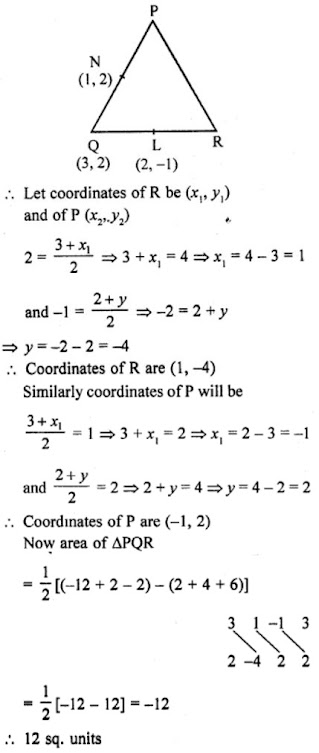9. If P (-5, -3), Q (-4, -6), R (2, -3) and S (1, 2) are the vertices of a quadrilateral PQRS, find its area.

Solution

P (-5, -3), Q (-4, -6), R (2, -3) and S (1,2) are the vertices of a quadrilateral PQRS.
Join PR which forms two triangles PQR and PSR.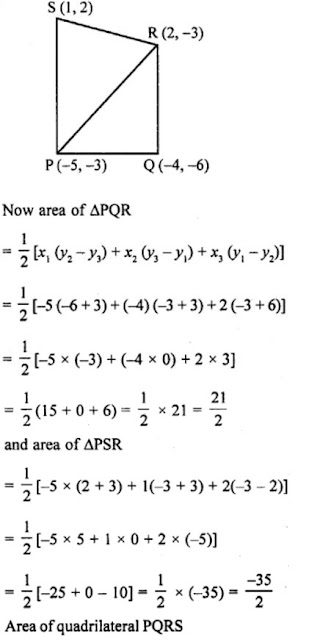10. If A (-3, 5), B (-2, -7), C (1, -8) and D (6, 3) are the vertices of a quadrilateral ABCD, find its area.

Solution

A (-3, 5), B (-2, -7), C (1,-8) and D (6, 3) are the vertices of a quadrilateral ABCD
Join AC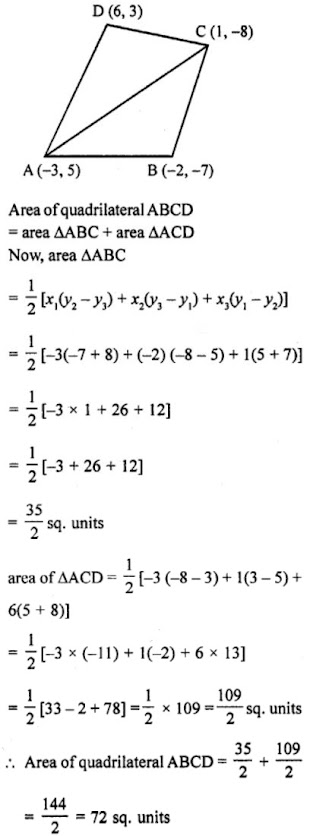11. For what value of ‘a’ the points (a, 1), (1, -1) and (11, 4) are collinear?

Solution

Let the vertices of ∆ABC are A (a, 1), B (1, -1) and C (11, 4)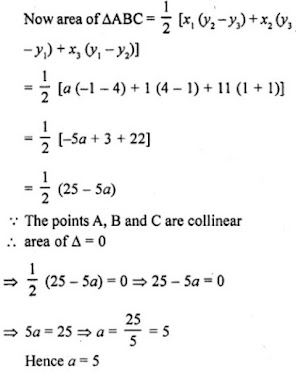12. Prove that the points (a, b), (a1, b1) and (a – a1, b – b1) are collinear if ab1 = a1b.

Solution

Let the points (a, b), (a1, b1) and (a – a1, b – b1) be the vertices of a triangle, then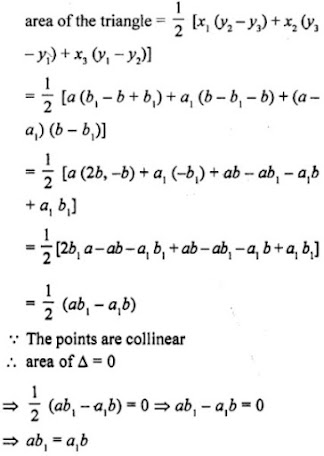13. If the vertices of a triangle are (1, -3), (4, p) and (-9, 7) and its area is 15 sq. units, find the value(s) of p.

Solution

The vertices of a triangle are (1, -3), (4, p) and (-9, 7) and area of triangle = 15 sq. units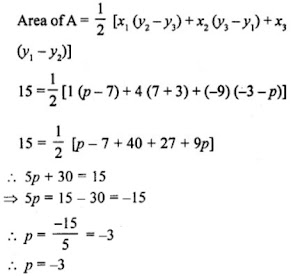14. If (x, y) be on the line joining the two points (1, -3) and (-4, 2), prove that x + y + 2 = 0.

Solution

Point (x, y) be on the line joining the two points (1, -3) and (-4, 2)
Points (x, y), (1, -3) and (-4, 2) are collinear
Let the points (x, y) (1, -3) and (-4, 2) are the vertices of a triangle, then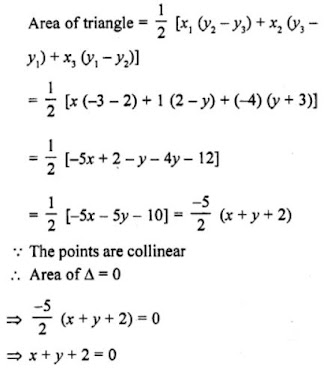15. Find the value of k if points (k, 3), (6, -2) and (-3, 4) are collinear.

Solution

Let the points (k, 3), (6, -2) and (-3, 4) be the vertices of a triangle, then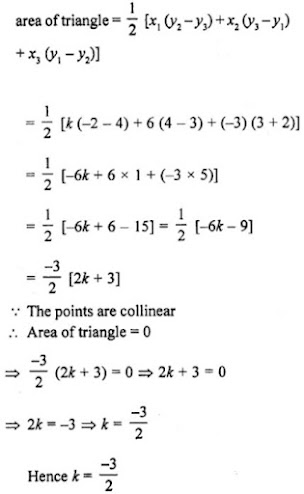16. Find the value of k, if the points A (7, -2), B (5, 1) and C (3, 2k) are collinear.

Solution

Points A (7, -2), B (5, 1) and C (3, 2k) are collinear
area of ∆ABC = 0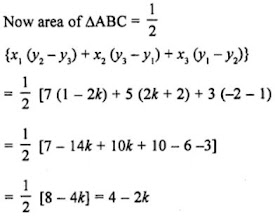∵ Points are collinear
∴ area of ∆ABC = 0
⇒ 4 - 2k = 0
⇒ 2k = 4
⇒ k = 2

17. If the point P (m, 3) lies on the line segment joining the points A (-2/5, 6) and B (2, 8), find the value of m.

Solution

The point P(m, 3) lies on the line segment joining the points A(-2/5, 6) and B(2, 8)
Points A, P, B are collinear.
Area of ∆APB = 0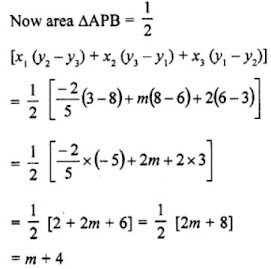∵ Area of ∆APB = 0
∴ m + 4 = 0
⇒ m = -4
Hence, m = -4